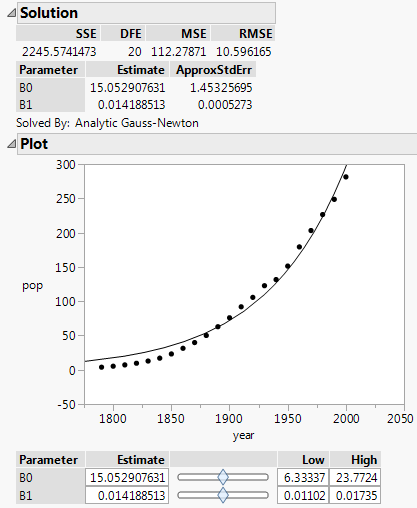Predictive and Specialized Modeling > Nonlinear Regression
Publication date: 11/10/2021

# Nonlinear Regression

##### Fit Custom Nonlinear Models to Your Data

The Nonlinear platform is a good choice for models that are nonlinear in the parameters. This chapter focuses on custom nonlinear models, which include a model formula and parameters to be estimated. Use the default least squares loss function or a custom loss function to fit models. The platform minimizes the sum of the loss function across the observations.

Figure 15.1 Example of a Custom Nonlinear FitThe Nonlinear platform also provides predefined models, such as polynomial, logistic, Gompertz, exponential, peak, and pharmacokinetic models. See Fit Curve.

Note: Some models are linear in the parameters (for example, a quadratic or other polynomial) or can be transformed to be such (for example, when you use a log transformation of x). The Fit Model or Fit Y by X platforms are more appropriate in these situations. For more information about these platforms, see Model Specification in Fitting Linear Models and Introduction to Fit Y by X in Basic Analysis.